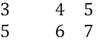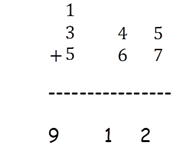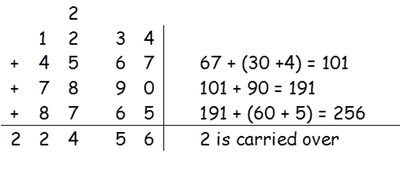This method is used to add big numbers which are greater than two digits.

Let’s learn this method by taking an example.
Let’s say we want to add 345 and 567.

First of all we are going to divide these numbers in double column.Here we have 45 + 67. So we can add it like 45 + (60 + 7)
This gives us 105 + 7.  And finally we have 112.
But the column is of only two digits.  So Left most ‘1’ is carried forward and ‘12’ is last two digits of the answer.

So now we haveAnother Example

Let’s say the numbers are 1234 + 4567+ 7890 + 8765.
Divide them into double column.This way you can add any numbers.